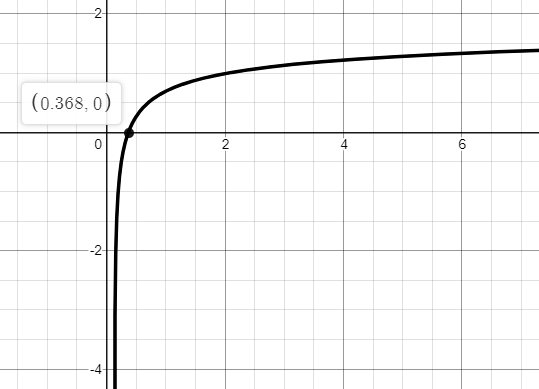# The domain of the function.### Single Variable Calculus: Concepts...

4th Edition
James Stewart
Publisher: Cengage Learning
ISBN: 9781337687805### Single Variable Calculus: Concepts...

4th Edition
James Stewart
Publisher: Cengage Learning
ISBN: 9781337687805

#### Solutions

Chapter 1.6, Problem 56E

(a)

To determine

## To find: The domain of the function.

Expert Solution

The domain of the function is x0.368 .

### Explanation of Solution

Given information:

The given function is,

f(x)=ln(2+lnx)

Consider the graph of the given function,From the graph, the domain of the given function is x0.368 .

(b)

To determine

### To find: The inverse and the domain of the inverse function.

Expert Solution

The inverse of the function is f1(x)=e(2ex) and the domain is all real numbers.

### Explanation of Solution

Given information:

The given function is,

f(x)=ln(2+lnx)

The inverse of the function is calculated as,

f(x)=ln(2+lnx)y=ln(2+lnx)ey=2+lnxlnx=2ey

Simplify further,

x=e(2ey)

For the inverse function, replace x and y ,

y=e(2ex)f1(x)=e(2ex)

Consider the graph of the inverse function,From the graph, the domain of the given function is 1.414x1.414 .

Thus, the inverse of the function is f1(x)=e(2ex) and the domain is all real numbers.

### Have a homework question?

Subscribe to bartleby learn! Ask subject matter experts 30 homework questions each month. Plus, you’ll have access to millions of step-by-step textbook answers!